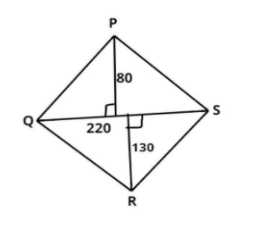Courses
Courses for Kids
Free study material
Free LIVE classes
MoreLIVE
Join Vedantu’s FREE Mastercalss

# Kalyan has a quadrilateral shaped field. If one of the diagonal of this farm is 220 m and length of perpendiculars from both the edges is 80 m and 130 m, then find the area of the field.Verified
364.8k+ views
Hint – In this question, first draw the diagram it will give us a clear picture of what we have to find out, then break the quadrilateral into two parts and calculate the area of these parts separately, so use this concept to reach the solution of the question.Let PQRS be a quadrilateral as shown above.
It is given that one of the diagonal of this farm is 220 m.
So let QS = 220 m.
Other edges of the quadrilateral are P and R.
And the length of perpendicular from both of the edges is 80 m and 130 m. as shown in figure.
Now as we see that QS is the common diagonal for both upper and lower triangles. (see figure)
So, the area of the quadrilateral is the sum of the areas of triangles.
$\Rightarrow {A_q} = {A_{U.t}} + {A_{L.t}}$
Where,
${A_q}$ = Area of quadrilateral.
${A_{U.t}}$ = Area of upper triangle.
${A_{L.t}}$ = Area of lower triangle.
Now as we know that the area of the triangle is half multiplied by base time’s perpendicular length.
So, first find out the area of the upper triangle.
${A_{U.t}} = \frac{1}{2}\left( {QS} \right)\left( {{\text{Perpendicular length}}} \right) = \frac{1}{2}\left( {220} \right)\left( {80} \right)$, (as perpendicular length for the upper triangle is 80 see figure)
Now, calculate the area of the lower triangle.
${A_{L.t}} = \frac{1}{2}\left( {QS} \right)\left( {{\text{Perpendicular length}}} \right) = \frac{1}{2}\left( {220} \right)\left( {130} \right)$, (as perpendicular length for the lower triangle is 130 see figure)
So, the area of quadrilateral is,
$\Rightarrow {A_q} = {A_{U.t}} + {A_{L.t}} \\ \Rightarrow {A_q} = \frac{1}{2}\left( {220} \right)\left( {80} \right) + \frac{1}{2}\left( {220} \right)\left( {130} \right) \\ \Rightarrow {A_q} = \frac{1}{2} \times 220 \times \left( {80 + 130} \right) \\ \Rightarrow {A_q} = 110 \times 210 = 23100{\text{ }}{{\text{m}}^2} \\$
So, the area of the quadrilateral field is 23100 sq. m

Note – Whenever we face such types of questions first draw the pictorial representation of the given problem then apply the formula of area of quadrilateral as stated above then substitute the values in this formula and simplify, we will get the required area of the field.

Last updated date: 29th Sep 2023
Total views: 364.8k
Views today: 7.64k